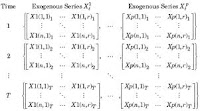## Saturday, February 16, 2013

### Advancing Matrix ArrayADVANCING MATRIX ARRAYS

A simple matrix is typically a rectangular array of elemental numbers. The Big Brain Machine Initiative takes this several levels deeper by creating the Advancing Matrix Array, which is an N-dimensional matrice that becomes "arrays of dimensions."

This can include the Calculus of space, time, and elements of relativity and beyond. Basic rules of the fundamental matrix is extended with transformations. For example, space is warped, time travel is divided, and relativistic holes are dimensionally added. Space is juxtaposed in the event of gravity lensing.

A matrix can control an array of LEDs. Perhaps more importantly, a matrix can control an array of space time and the elements of relativity. This type of matrice could control relativity space time flux for a time travel pod, or it could serve as the manifold tuner in a matter-antimatter chamber.

It can also be used to control Universe gates and doorways. It could help open a portal to a new place in space and time. It could serve as the navigational control in the shifting proximity of space altered by gravity wells. Perhaps it could help a machine probe circumnavigate the outer fringes of Black Holes to gain a greater understanding into their relativistic jets.Beginning a Matrix Array experiment
Advancing Matrix Arrays have many uses for the insides of massive Transposition Machines by controlling, setting, creating, and transforming their internal parameters and NDIMS.

The AMAs work well in the technology of MIMs, machines in machines, and transforming not only matrices but entire machines. Another example is in the performance of JAVA design computational tools. Referring to the matrix 3 diagram, in the example of Java computational design tools, the 3 dimensional array of integer in the code can be diagrammed like the figure. A 3 dimensional array is an array of arrays of array. The length of the first dimension is matrix3.length. The length of the second dimension is matrix.length. The length of the third dimension is matrix.length. Like 2 dimensional arrays, this 3 dimensional array also has matrix.length , matrix.length , matrix.length , matrix.length , matrix.length and matrix.length independently, but again they are all same value in this code. (see http://igeo.jp/tutorial/6.html)